102
Exact String Matching Algorithms
String-algorithm

Preliminary Definitions

A string is a sequence of characters. In our model we are going to represent a string as a 0-indexed array. So a string S = ”Galois” is indeed an array [‘G’, ’a’, ’l’, ’o’, ’i’, ’s’]. The number of characters of a string is called its length and is denoted by |S|. If we want to reference the character of the string at position i, we will use S[i].

A substring is a sequence of consecutive contiguous elements of a string, we will denote the substring starting at i and ending at j of string S by S[i...j].

A prefix of a string S is a substring that starts at position 0, and a suffix a substring that ends at |S|-1. A proper prefix of a S is a prefix that is different to S. Similarly, a proper suffix of S is a suffix that is different to S. The + operator will represent string concatenation.

Example:

S="Galois"
|S|=6
S='G', S='a', S='l',...,S='s'
S[1...4]="aloi"
W="Evariste"
W+S="EvaristeGalois"


A Needle in the haystack (KMP algorithm)

Given a text T we are interested in calculating all the occurrences of a pattern P.

This simple problem has a lot of applications. For example, the text can be the nucleotide sequence of the human DNA and the pattern a genomic sequence of some genetic disease, or the text can be all the internet, and the pattern a query (the Google problem), etc.

We are going to study the exact string matching problem, that is given two strings T and P we want to find all substrings of T that are equal to P. Formally, we want to calculate all indices i such that T[i+s] = P[s] for each 0 ≤ s ≤ |P|-1.

In the following example, you are given a string T and a pattern P, and all the occurrences of P in T are highlighted in red.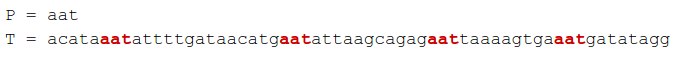One easy way to solve the problem, is to iterate over all i from 0to |T| - |P|, and check if there is a substring of T that starts at i and matches with P:

def find_occurrences(t,p):
lt,lp=len(t),len(p)
for i in range(lt-lp+1):
match=True
for l in range(lp):
if t[i+l]!=p[l]:
match=False
break
if match: print i


Unfortunately this solution is very slow, its time complexity is O(|T|·|P|), however it gives us some insights.

Suppose that we are finding a match starting at position i on the text, then there are two possibilities:

We don’t find a match. Then there exists at least one index in which the text is not equal to the pattern. Let i+j be the smallest of such indices: T[i...i+j-1] = P[0...j-1] and T[i+j] ≠ P[j]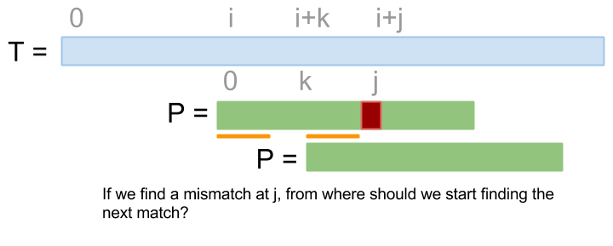Since there is no match at position i, we should start finding a match from another position, but the question is from where? Our previous algorithm always selects as next position i+1, but if we start from i+1, it is probable that we could end up finding a mismatch in a position even before than i+j-1. At least, we could want to start from a position that guarantees that the strings matches until i+j-1. Therefore, we should start finding for a match starting from the smallest i+k such that T[i+k...i+j-1] matches with some prefix of P (look at the picture above).

Since we already know that T matches with P from i to i+j-1, then T[i+k...i+j-1] is a suffix of P[0...j-1].

That means that if we find a mismatch at position j, we should start from the smallest k such that P[k...j-1] is a prefix of P. k is the smallest, so P[k...j-1] is the largest proper suffix that is also a proper prefix. From now on we will call “border” to the proper prefixes that are also proper suffixes (e.g the string ABCDABCDAB have two borders ABCDAB and AB).

We find a match. Using the same argument, it’s easy to see that we have to start finding for a match from the smallest k such that P[k...j-1]is a proper prefix of P

Let’s see the above two cases with an example: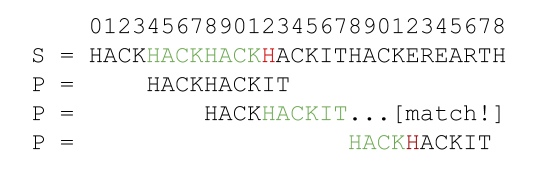In the picture above we are finding occurrences starting from position 4, but there is a mismatch at position 12. Before getting a mismatch we have already matched the string HACKHACK, and the largest proper prefix that is also a proper suffix (border) of HACKHACK is HACK, so we should start finding occurrences again from position 8 and taking into account that all characters from 8 to 11 are already matched. It turns out that there is an occurrence starting at position 8, so all characters of the pattern have matched, since HACKHACKIT does not have any border, then we start finding occurrences again starting from position 18.

According to the previous analysis we need to know for each i, the the largest border of P[0...i]. Let f[i] be the length of the largest border of P[0...i]. Function f is known as failure function, because it says from where start if we find a mismatch.

How can we calculate f[i] efficiently? One approach is to think in an inductive way: suppose that we have already calculated the function f for for all indices less than i. Using that information how can we calculate f[i]?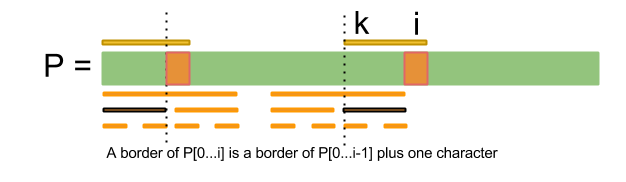Note that if P[0...j] is a border of P[0...i], then P[0...j-1] is a border of P[0...i-1] and P[j] = P[i].

The previous argument suggest this algorithm for finding f[i]: Iterate over all borders of P[0...i-1], in decreasing order of length, until find a border P[0...j-1] such that P[j] = P[i]. How can we iterate over all the borders of a string? That can be easily done using this observation: if B is a border of P, then a border of B is also a border of P, that implies that the borders of P[0...i] are prefixes of P that ends at f[i]-1, f[f[i]-1]-1, f[f[...f[i]-1...]-1]-1, ... (at most how many borders can have a string?).

What is the complexity of our algorithm? Let P[j...i-1] be the largest border of P[0...i-1]. If P[k...i] is the largest border of P[0...i], then k ≥ j (Why?). So when we iterate over the borders of P[0...i-1], we are moving the index j to k. Since j is moving always to the right, in the worst case it will touch all the elements of the array. That means that we are calculating function f in O(|P|).

Using function f it is easy to search for the occurrences of a pattern on a text in O(|T|+|P|):

def failure_function(p):
l=len(p)
f=*l
j=0
for i in range(1,l):
while j>=0 and p[j]!=p[i]:
if j-1>=0: j=f[j-1]
else: j=-1
j+=1
f[i]=j
return f

def find_occurrences(t,p):
f=failure_function(p)
lt,lp=len(t),len(p)
j=0
for i in range(lt):
while j>=0 and t[i]!=p[j]:
if j-1>=0: j=f[j-1]
else: j=-1
j+=1
if j==lp:
j=f[lp-1]
print i-lp+1


As you can note from the pseudo code (it is python code indeed), find_occurrences is almost equal to failure_function, that is because in some sense failure_function is like matching a string with itself.

The algorithm described above is known as Knut-Morris-Pratt (or KMP for short). Note that with KMP algorithm we don’t need to have all the string T in memory, we can read it character by character, and determine all the occurrences of a pattern P in an online way.

The Z function

Given a string S, let z[i] be the longest substring of S that starts at i and is also a prefix of S.

Example: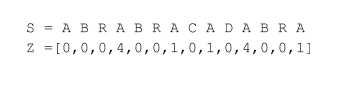z[ 3 ] = 4 because starting from position 3, the largest string that is also a prefix of S is ABRA.

If we can calculate the function Z efficiently, then how can we find all the occurrences of a pattern P on a text T? Since z[i] gives matches with a prefix, a good idea is to observe how the z function behaves when P is concatenated with T. So let’s calculate the function z on S = P+T. It turns out that if for certain i, z[i] ≥ |P| then there is an occurrence of P starting at i.

Now only remains to find a way of calculate that powerful z function.

The naive approach for calculate z in every i is to iterate over all j ≥ i until we find a mismatch ( z[i...j] ≠ z[0...j-i] ), then z[i] = j-i. This algorithm is of quadratic time, so we need a better solution.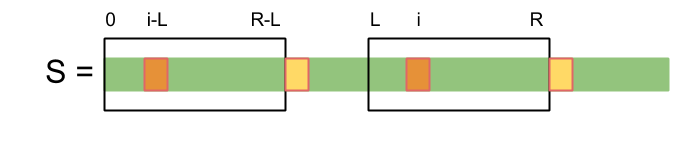The z function applied at position i of a string determines an interval [i, i+z[i]-1] known as z-box, that interval is special, because by the definition of z, S[ i...i+z[i]-1 ] = S[0...z[i]-1].

Now let’s think again in a inductive way, and calculate z[i] given that we already know z,...,z[i-1].

Let [L,R] be the z-box with largest R that we have seen until now.

If i is inside [L,R], then S[i...R] = S[i-L...R-L] (look at the picture above), so we can use the value of z[i-L] that we have already calculated. If z[i-L] Is less than R-i+1, then z[i] = z[i-L], otherwise since we already know that S[i...R] is a prefix of S, it remains to check if we can expand S[i...R] starting from R+1.

On the other hand If i is outside [L...R], then we can calculate z[i] using the naive approach. This algorithm is linear because the pointer R traverses the array at most once.

def zeta(s):
n=len(s)
z=*n
L,R=-1,-1
for i in range(1,n):
j,k=0,i
if L<=i<=R:
ii = i-L
j=min(ii+z[ii], R-L+1)-ii
k=i+j
while k<n and s[j]==s[k]:
j+=1
k+=1
z[i]=k-i
if z[i]>0 and i+z[i]-1>R:
L,R=i,i+z[i]-1
return z

def find_occurrences(p,t):
lp,lt=len(p),len(t)
z=zeta(p+t)
for i in range(lp,lp+lt):
if z[i]>=lp: print i-lp


Hashing strikes back (Rabin-Karp algorithm)

Pattern P matches with text T at position i, if and only if there is a substring of T that starts at i and is equal to P. So if we can compare quickly two strings ( T[i...i +|P|-1] with P ), then we can use our naive algorithm (iterate over all i, and check if there is a match). One way of checking if two strings are not equal is to find a property that is different in those strings. Let h be a black-box that have as input a string, and outputs certain property of the string, that property is defined in such a way that if two strings are different, the value of that property is also different: if S1 ≠ S2 then h(S1) ≠ h(S2). Note that with this definition, we can’t assert if two strings are equal, because two different strings can have the same value of the chosen property.

Since numbers are easy to compare it will be nice if h outputs a number. So the question is how to represent a string as a number. Note that strings are like numbers, because we can consider its characters as digits. Since the alphabet have 26 letters, we could say that a string is a number in a numeration system of base 27. The problem is that strings can be very large, and we can represent integers in a very limited range (from 0 to 18446744073709551615 using a 64 bit unsigned integer), in order to keep the output of h in a small range, let’s apply the modulo operation (is because of this last necessary operation that two different strings can map to the same integer i.e there is a hash collision).

Example: if S=”hack”, then h(S) = (8 · 283 + 1 · 282 + 3 · 281 + 11 · 280) %M.

Note that we are considering the value of the digit “a” as 1 instead of 0, that is to prevent cases with leading zeroes (e.g “aab” and “b” are different, but if a = 0 they are equal).

A function like h, that converts elements from a very large range (like strings) to elements in a small range (64 bit integers) are called hash functions The value of the hash function applied to certain element is called it’s hash.

In order to reduce hash collisions, M is usually chosen as a large prime. However if we use an unsigned 32 bits integer we could just let the value overflow (in this case the modulo is 232).

It remains to solve this problem: if we know the hash of the substring (of length |P|) that starts at i, how to calculate the hash of the substring that starts at i+1? (see figure below)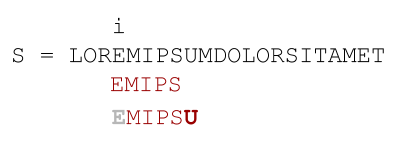Let h be the hash of the substring of length |P| that starts at i. We can calculate the hash of the substring that starts at i+1 in two steps:

Remove the character at position i: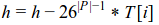Add the character at position i+|P|: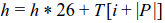The idea of calculate the hash that starts at position i+1 using the hash at i is called rolling hash.

def val(ch): #maps a character to a digit e.g a = 1, b = 2,...
return ord(ch)-ord("a")+1

def find_occurrences(p,t):
lp,lt=len(p),len(t)
m=10**9+7 #modulo (a "big" prime)
b=30      #numeration system base
hp=0      #hash of the pattern
ht=0      #hash of a substring of length |P| of the text
for i in range(lp):
hp=(hp*b+val(p[i]))%m
ht=(ht*b+val(t[i]))%m
pwr=1
for i in range(lp-1):
pwr=pwr*b%m

if hp==ht: print 0
for i in range(1,lt-lp+1):
#rolling hash
#remove character i-1:
ht=(ht-val(t[i-1])*pwr)%m
ht=(ht+m)%m